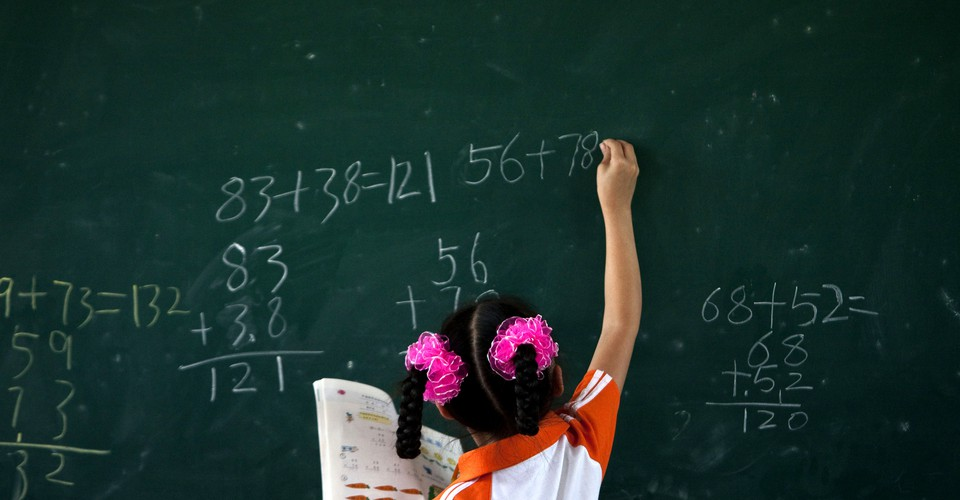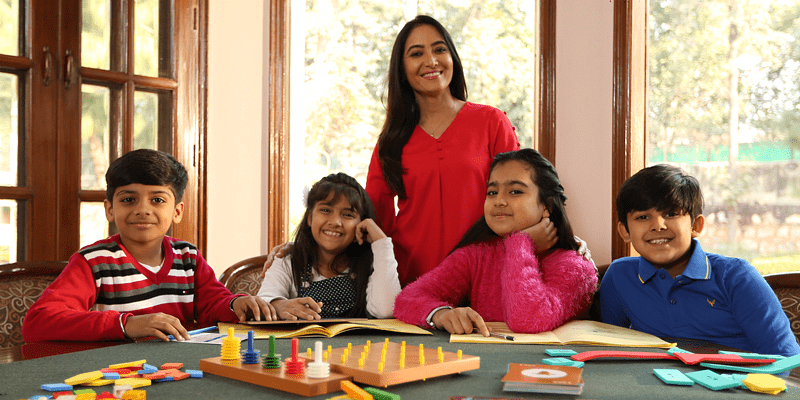# Cuemath Vs Abacus, the best Math program Analysis

45 views

 1 Introduction 2 Abacus-Faster Arithmetic Calculations 3 Cuemath – Learn Math By Reasoning 4 Comparison of Abacus vs Cuemath 5 Abacus vs Cuemath – Summary 6 FAQs

## Introduction

Both Cuemath and Abacus are after-school Math programs. But the focus of these programs is on very different aspects of math. Depending on which aspect you feel is right for you, the decision-making process could be made easier by this analysis put together by the Cuemath Team.

## Abacus – Faster Arithmetic Calculations

Abacus helps children aged 4 to 13, calculate faster. It is designed to increase the speed of mental calculations. The program has different levels and students proceed through these levels by learning to mentally calculate increasingly difficult arithmetic problems. It helps children with all four arithmetic operations – addition, subtraction, multiplication, and division.At the core of the Abacus, a learning methodology is a calculation tool used for thousands of years – the abacus. Initially, students start by using physical abacus to perform calculations.

Then students are trained to remember visual pictures of the abacus in their minds to help them calculate faster. By the end of the Abacus program, children would have memorized 450,000 abacus images.

They use these images to help them calculate fast and mentally. Thus as a parent, if your objective is to make your child faster at arithmetic calculations, then the Abacus program might work for you.

## Cuemath – Learn Math By Reasoning

Cuemath is a math program for children aged 5 to 16. Cuemath focuses on building a strong math foundation and helping children learn conceptually instead of through memorization.

It not only focuses on arithmetic but on all math concepts that children learn at school. Children learn by solving problems that require them to reason and figure things out.

At the core of the Cuemath learning philosophy is the Cuemath Method. A core principle of this method is learning by reasoning. Cuemath helps children develop their ability to analyze and solve complex problems through reasoning.

Another core principle is conceptual learning by introducing every math concept either via an activity or through pictorial models. Then, Cuemath teachers are trained to help students by not giving the answers away.

Instead, they cue the answer by asking leading questions, giving hints so that students discover concepts. All students work on their own learning resources allowing them to speed up or slow down based on their comfort with the topic.Along with this focus on the basics of concepts, students also get sufficient practice to ensure they solve questions efficiently.

Apart from this, students are assigned skill-building exercises on the tab in each class. They are also assigned a math and logic puzzle at the end of every class.

As a parent, if your objective is a strong math foundation across all topics and not just arithmetic, then the Cuemath program is a better choice.

## Comparison of Abacus vs Cuemath

 Abacus Cuemath Age group 4 to 13-year-olds 5 to 16-year-olds Math topics covered Arithmetic operations All topics covered by the school Batch sizes 15 students per batch 6 students per batch Math learning aids Abacus Abacus, Base-10 blocks, Fraction shapes, Geo solids, Pattern blocks, and 10 more. Aptitude exercises No Yes, every class. Math and logic puzzles No Yes, every class. Core philosophy Add, subtract, multiply and divide faster by memorizing thousands of abacus images. Learn by reasoning and build a solid math foundation. Know the reason behind the steps.

## Abacus vs Cuemath – Summary

Calculating faster is an impressive skill, however, it is increasingly an outdated skill. Today each one of us carries a powerful smartphone that can perform calculations for us.

What is needed today is the ability to figure out how to solve complex unsolved problems. This requires reasoning and analytical skills.

Given a data set and a problem, knowing if one needs to calculate the mean or the median is important. Once this decision is taken any smartphone can calculate the value in an instant.

So students must learn to make good decisions rather than calculate faster. This is where a program like Cuemath is more helpful and will help prepare students for the future.

## What is the difference between Cuemath and Vedic Math?

Vedic math has increased in popularity in recent years. It is a collection of techniques/sutras to solve mathematical problem-sets in a fast and easy way. Here’s a short article to help you understand what Vedic Math offers and how it compares with Cuemath.

## Cuemath vs Abacus - Which one is better?

Both Cuemath and Abacus are after-school Math programs. But the focus of these programs is on very different aspects of math. The decision becomes clear when you understand what is right for your child.

## Is Cuemath good?

At Cuemath, children learn by understanding the reason and logic behind every math problem before attempting to arrive at any solutions. This is backed by our unique methodology of teaching the 'Why before the What' in math concepts.

## What is Cuemath’s philosophy/ vision?

Our vision is to create thinkers and problem solvers of tomorrow. A robust math foundation will help children overcome the fear of math and discover the fascinating side of it. We approach math as a life skill and want children to be math literate and not view math as just a school subject. Our curriculum has been designed by math experts with a strong focus on building mental aptitude and creative reasoning while covering school curriculum.

## What is the Cuemath curriculum / learning methodology? What all does it cover and how is it delivered?

A child is considered good at math if they score well in school exams. However, it's not an accurate indicator of a child’s math ability. Many students score 95% to 100% in their exams, but are not clear about key math concepts. It is important to note that a weak math foundation becomes evident in higher grades when a child finds it difficult to deal with increasing complexity. At Cuemath, we give personal attention to your child to analyse their improvement areas. For students who are already strong in fundamentals, our program focuses on advanced concepts (with increasing difficulty level, Olympiad preparation, etc). Furthermore, children who are good at math might lose interest in the subject because their schools may not challenge them enough to keep them engaged. At Cuemath, students are constantly challenged with tab-based activities and puzzle cards among others.

Related Articles
GIVE YOUR CHILD THE CUEMATH EDGE
Access Personalised Math learning through interactive worksheets, gamified concepts and grade-wise courses